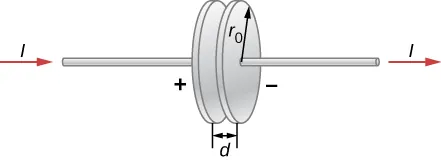University Physics Volume 2

# Challenge Problems

University Physics Volume 2Challenge Problems

### Challenge Problems

106.

A parallel-plate capacitor with plate separation d is connected to a source of emf that places a time-dependent voltage V(t) across its circular plates of radius $r0r0$ and area $A=πr02A=πr02$ (see below).(a) Write an expression for the time rate of change of energy inside the capacitor in terms of V(t) and dV(t)/dt.

(b) Assuming that V(t) is increasing with time, identify the directions of the electric field lines inside the capacitor and of the magnetic field lines at the edge of the region between the plates, and then the direction of the Poynting vector $S→S→$ at this location.

(c) Obtain expressions for the time dependence of E(t), for B(t) from the displacement current, and for the magnitude of the Poynting vector at the edge of the region between the plates.

(d) From $S→S→$, obtain an expression in terms of V(t) and dV(t)/dt for the rate at which electromagnetic field energy enters the region between the plates.

(e) Compare the results of parts (a) and (d) and explain the relationship between them.

107.

A particle of cosmic dust has a density $ρ=2.0g/cm3.ρ=2.0g/cm3.$ (a) Assuming the dust particles are spherical and light absorbing, and are at the same distance as Earth from the Sun, determine the particle size for which radiation pressure from sunlight is equal to the Sun’s force of gravity on the dust particle. (b) Explain how the forces compare if the particle radius is smaller. (c) Explain what this implies about the sizes of dust particle likely to be present in the inner solar system compared with outside the Oort cloud.

Order a print copy

As an Amazon Associate we earn from qualifying purchases.